# ML Aggarwal Class 8 Solutions for ICSE Maths Chapter 18 Mensuration Ex 18.4

## ML Aggarwal Class 8 Solutions for ICSE Maths Chapter 18 Mensuration Ex 18.4

Question 1.
The surface area of a cube is 384 cm2. Find
(i) the length of an edge
(ii) volume of the cube.
Solution:Question 2.
Find the total surface area of a solid cylinder of radius 5 cm and height 10 cm. Leave your answer in terms of n.
Solution: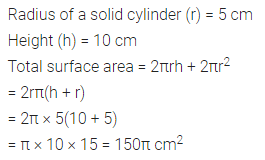Question 3.
An aquarium is in the form of a cuboid whose external measures are 70 cm × 28 cm × 35 cm. The base, side faces and back face are to be covered with coloured paper. Find the area of the paper needed.
Solution:Question 4.
The internal dimensions of the rectangular hall are 15 m × 12 m × 4 m. There are 4 windows each of dimension 2 m × 1.5 m and 2 doors each of dimension 1.5 m × 2.5 m. Find the cost of whitewashing all four walls of the hall, if the cost of whitewashing is ₹5 per m2. What will be the cost of whitewashing if the ceiling of the hall is also whitewashed?
Solution:Question 5.
A swimming pool is 50 m in length, 30 m in breadth and 2·5 m in depth. Find the cost of cementing its floor and walls at the rate of ₹27 per square metre.
Solution: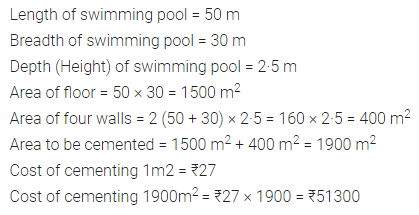Question 6.
The floor of a rectangular hall has a perimeter 236 m. Its height is 4·5 m. Find the cost of painting its four walls (doors and windows be ignored) at the rate of Rs. 8.40 per square metre.
Solution: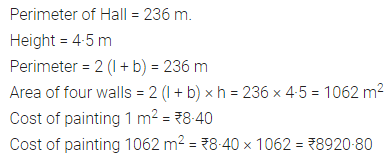Question 7.
A cuboidal fish tank has a length of 30 cm, a breadth of 20 cm and a height of 20 cm. The tank is placed on a horizontal table and it is three-quarters full of water. Find the area of the tank which is in contact with water.
Solution: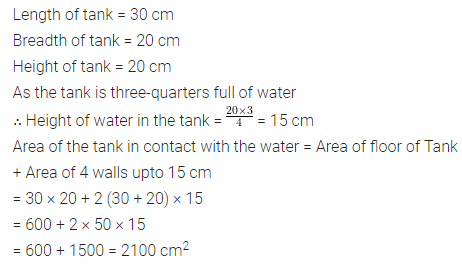Question 8.
The volume of a cuboid is 448 cm3. Its height is 7 cm and the base is a square. Find
(i) a side of the square base
(ii) surface area of the cuboid.
Solution: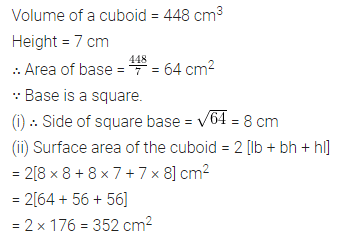Question 9.
The length, breadth and height of a rectangular solid are in the ratio 5 : 4 : 2. If its total surface area is 1216 cm2, find the volume of the solid.
Solution:Question 10.
A rectangular room is 6 m long, 5 m wide and 3·5 m high. It has 2 doors of size 1·1 m by 2 m and 3 windows of size 1·5 m by 1·4 m. Find the cost of whitewashing the walls and the ceiling of the room at the rate of ₹5·30 per square metre.
Solution: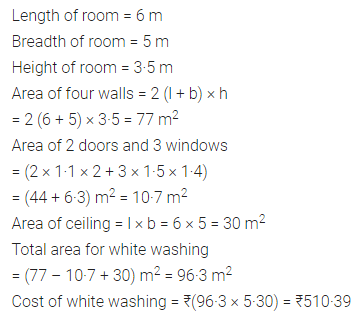Question 11.
A cuboidal block of metal has dimensions 36 cm by 32 cm by 0·25 m. It is melted and recast into cubes with an edge of 4 cm.
(i) How many such cubes can be made?
(ii) What is the cost of silver coating the surfaces of the cubes at the rate of ₹0·75 per square centimetre?
Solution: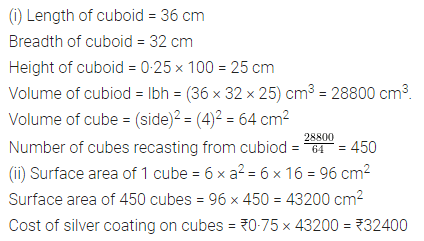Question 12.
Three cubes of silver with edges 3 cm, 4 cm and 5 cm are melted and recast into a single cube, find the cost of coating the surface of the new cube with gold at the rate of ₹3·50 per square centimetre?
Solution: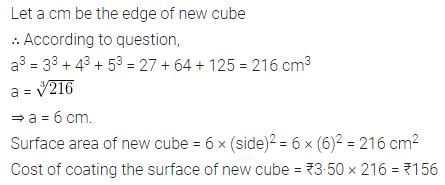Question 13.
The curved surface area of a hollow cylinder is 4375 cm2, it is cut along its height and formed a rectangular sheet of width 35 cm. Find the perimeter of the rectangular sheet.
Solution: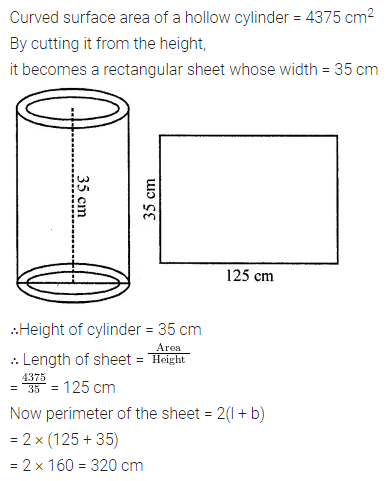Question 14.
A road roller has a diameter of 0.7 m and its width is 1.2 m. Find the least number of revolutions that the roller must take in order to level a playground of size 120 m × 44 m.
Solution:Question 15.
A company packages its milk powder in cylindrical container whose base has a diameter of 14 cm and height 20 cm. Company places a label around the surface of the container (as shown in the figure). If the label is placed 2 cm from top and bottom, what is the area of the label?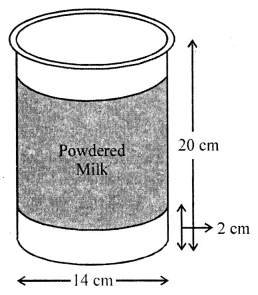Solution: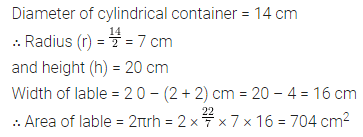Question 16.
The sum of the radius and height of a cylinder is 37 cm and the total surface area of the cylinder is 1628 cm2. Find the height and the volume of the cylinder.
Solution:Question 17.
The ratio between the curved surface and total surface of a cylinder is 1 : 2. Find the volume of the cylinder, given that its total surface area is 616 cm3.
Solution:Question 18.
The given figure showed a metal pipe 77 cm long. The inner diameter of the cross-section is 4 cm and the outer one is 4.4 cm.
Find its
(i) inner curved surface area
(ii) outer curved surface area
(iii) total surface area.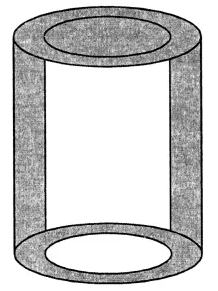Solution: